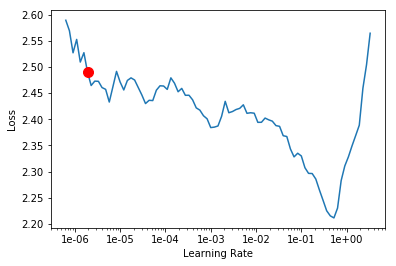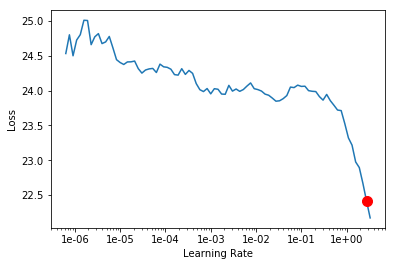# Collaborative filtering from scratch

Hi guys,
I’m trying to implement my own version of EmbeddingDotBias and EmbeddingNN to try to create a recommendation system for biological applications. For instance, I have 45854 users and 488 items. At first I thought this was going to be very straightforward, so I just copy-pasted the source code from here and tried to run it. What was my surprise when I got an error!

``````RuntimeError: index out of range at /opt/conda/conda-bld/pytorch-cpu_1556653093101/work/aten/src/TH/generic/THTensorEvenMoreMath.cpp:193
``````

That was quite interesting since it was supposed to run smoothly (it’s the same model as you get by running:

``````collab_learner(data,n_factors,wd)
``````

And here’s the custom model arch

``````copypaste_dotbias(
(u_weight): Embedding(45854, 50)
(i_weight): Embedding(488, 50)
(u_bias): Embedding(45854, 1)
(i_bias): Embedding(488, 1)
)
``````

As you can see the embedding sizes are what we expected, with the number of rows equals to number of users or items and each column representing a latent factor. But what if we create a collab_learner the same way Jeremy did on Lessons 4 and 5?

``````    learn_dot = collab_learner(data,n_factors=50,wd=1e-1,y_range=y_range,metrics=root_mean_squared_error)
``````

And when I print the arch:

``````print(learn_dot.model)

EmbeddingDotBias(
(u_weight): Embedding(39080, 50)
(i_weight): Embedding(489, 50)
(u_bias): Embedding(39080, 1)
(i_bias): Embedding(489, 1)
)
``````

As you can see the embeddings ARE different. For instance, the number of users is considerably smaller and the items embedding has an additional row. What is happening? Well, I still haven’t figured out but searching on forums I found threads like this and this one showing that fast.ai actually adds a NaN entry on the items embedding, which corresponds to our additional row. But what about the lower number of users? I don’t know, so if you already solved this please post hereI managed to train my custom model but I had to add 1 to BOTH embeddings and ALSO to my bias, so the dimensions match when the forward step is called. Here’s the code:

``````class myDotBias(nn.Module):
def __init__(self,nmols,ntargets,emb_size,y_range):
super().__init__()
self.mols_w = nn.Embedding(nmols+1,emb_size)
self.targets_w = nn.Embedding(ntargets+1,emb_size)
self.mols_b = nn.Embedding(nmols+1,1)
self.targets_b = nn.Embedding(ntargets+1,1)
self.max_range = y_range
self.min_range = y_range

def forward(self,m,t):
dot = self.mols_w(m)*self.targets_w(t)
res = dot.sum(1) + self.mols_b(m).squeeze() + self.targets_b(t).squeeze()
out = torch.sigmoid(res) * (self.max_range-self.min_range) + self.min_range
return out
``````

As you can see this is basically the same code as EmbeddingDotBias and it runs just OK.
Except it doesn’t! If you run both EmbeddingDotBias and myDotBias you get VERY different RMSE. For instance, lr_find() returned different ranges and shapes for the loss function surface.

Fastai EmbeddingDotBiasCustom EmbeddingDotBiasAs you can see, the loss values for the custom model are approx. 10x higher than fast.ai implementation. But why is that? The first thing that came to my mind was to check how the embeddings were created. If you take a closer look at myDotBias class you will see that the embeddings were randomly created by calling `nn.Embedding`.
On the other hand, fastai EmbeddingDotBias uses a different function called `embedding`. If you look at the source code of `embedding` you will see there is a reference to a paper called An Exploration of Word Embedding Initialization in Deep-Learning Tasks. The authors argue that there isn’t much different on how the embeddings are generated as long as the standard deviation is low (e.g. lower than 0.1). If we check the source code of the embedding function:

``````embedding??

Signature: embedding(ni:int, nf:int) -> torch.nn.modules.module.Module
Source:
def embedding(ni:int,nf:int) -> nn.Module:
"Create an embedding layer."
emb = nn.Embedding(ni, nf)
# See https://arxiv.org/abs/1711.09160
return emb
``````

It actually uses a very low standard deviation of 0.01. But what about Pytorch nn.Embedding? I couldn’t figure out yet, but I think it generates a different distribution with higher standard deviation.

Just to summarize this I found out 2 tweaks to make your custom collaborative filtering model: